# 为什么你学不会递归？谈谈我的经验# 前言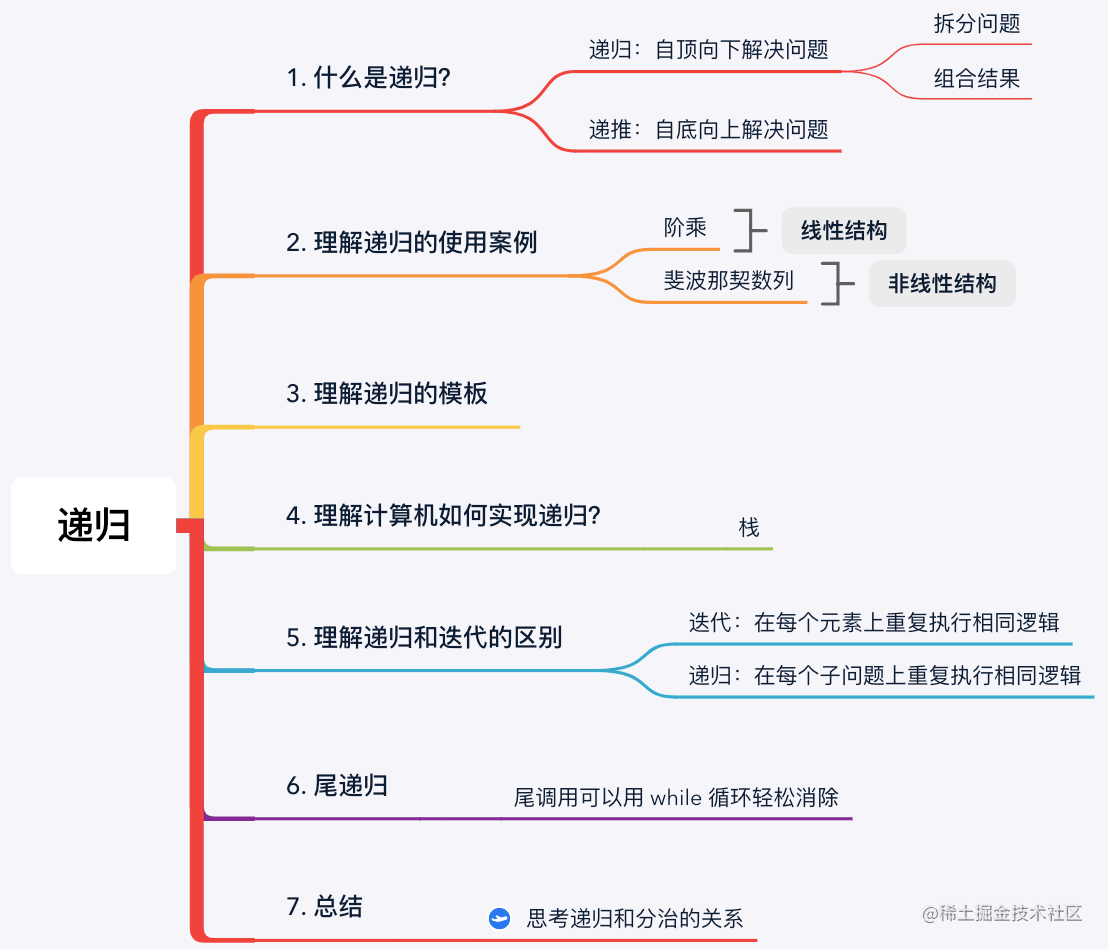# 1. 什么是递归？

• 递推（一般思维）： 我们从 $1$ 开始，用 $1$ 乘以 $2$ 得到 $2!$ 问题的解，用 $3$ 乘以 $2!$ 得到 $3!$ 问题的解。依次类推，直到用 $n$ 乘以 $(n-1)!$ 得到原问题 $n!$ 的解。这就是用递推解决问题，这是相对简单直接的思考方式；
• 递归（计算机思维）： 我们把 $n!$ 的问题拆分为一个 $(n-1)!$ 的问题，如果我们知道 $(n-1)!$ 的解，那么将它乘以 $n$ 就可以得出 $n!$ 的解。以此类推，我们将一个 $(n-1)!$ 的问题拆分为同类型的规模更小的 $(n-2)!$ 子问题，直到拆分到无法拆分，可以直接得出结果 $1!$ 问题。此时，我们再沿着拆分问题的路径，反向地根据子问题的解求出原问题的解，最终得到原问题 $n!$ 的结果。这就是用递归解决问题。

求 n!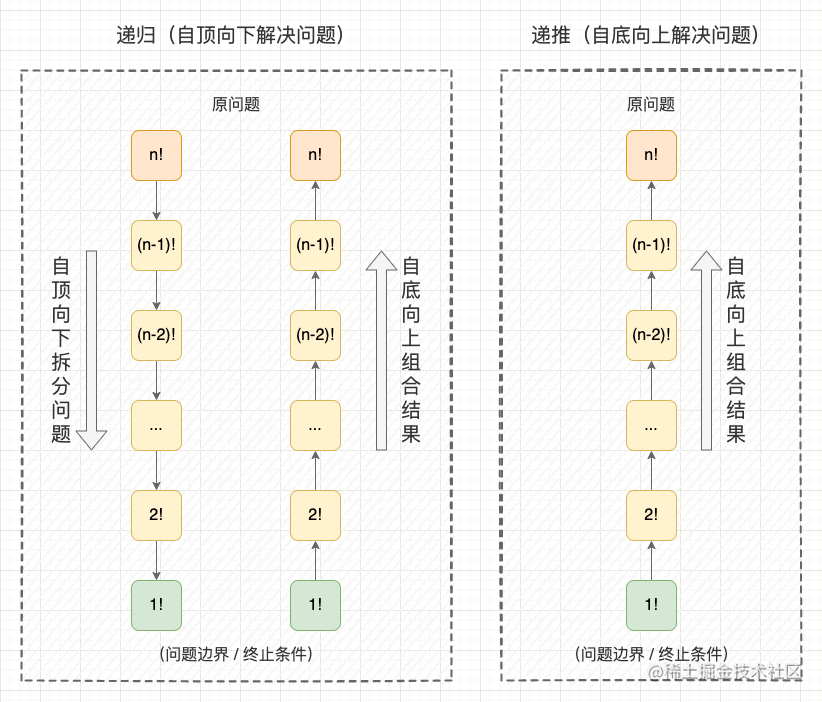• 1、自顶向下拆分问题： 从一个很难直接求出结果的、规模较大的原问题开始，逐渐向下拆分为规模较小的子问题（从 $n!$ 拆分到 $(n-1)!$），直到拆分到问题边界时停止拆分，这个拆分的过程就是 “递”（问题边界也叫基准情况或终止条件）；
• 2、自底向上组合结果： 从问题边界开始，逐渐向上传递并组合子问题的解（从 $(n-1)!$ 得到 $n!$），直到最终回到原问题获得结果，这个组合的过程就是 “归”。

LeetCode 例题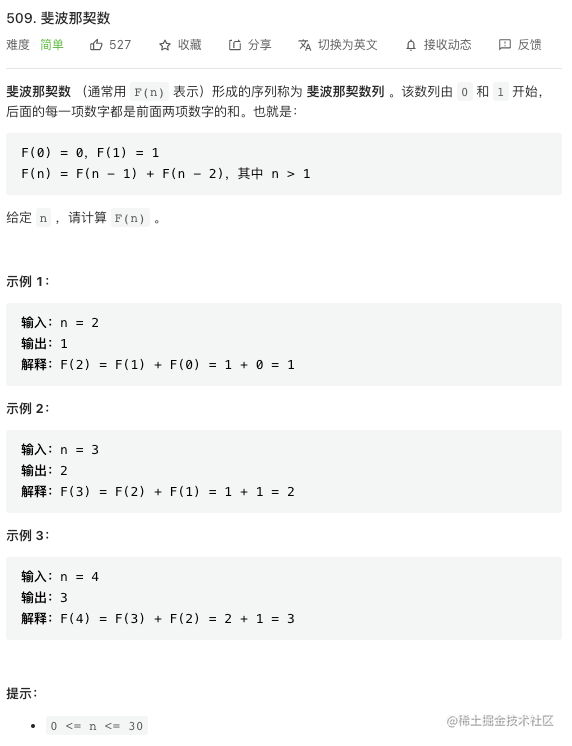用递归解决斐波那契数列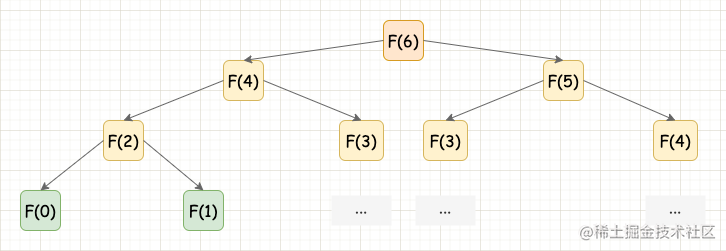用递归解决（无优化）

class Solution {
fun fib(N: Int): Int {
if(N == 0){
return 0
}
if(N == 1){
return 1
}
// 拆分问题 + 组合结果
return fib(N - 1) + fib(N - 2)
}
}

# 2. 递归的解题模板

• 1、判断当前状态是否异常，例如数组越界，n < 0 等；
• 2、判断当前状态是否满足终止条件，即达到问题边界，可以直接求出结果；
• 3、递归地拆分问题，缩小问题规模；
• 4、组合子问题的解，结合当前状态得出最终解。
fun func(n){
// 1. 判断是否处于异常条件
if(/* 异常条件 */){
return
}
// 2. 判断是否满足终止条件（问题边界）
if(/* 终止条件 */){
return result
}
// 3. 拆分问题
result1 = func(n1)
result2 = func(n2)
...
// 4. 组合结果
return combine(result1, result2, ...)
}

# 3. 计算机如何实现递归？

• 拆分问题： 就是一次子问题入栈的过程；
• 组合结果： 就是一次子问题出栈的过程。

• 调用函数： 程序会创建一个新的栈帧并压入调用栈的顶部；
• 函数返回： 程序会将当前栈帧从调用栈栈顶弹出，并带着返回值回到上一层栈帧中调用函数的位置。

# 4. 递归与迭代的区别

• 迭代： 通过迭代器（for/while）重复执行某一段逻辑；
• 递归： 通过函数自调用重复执行函数中的一段逻辑。

• 迭代：迭代的思路认为只要从问题边界开始，在所有元素上重复执行相同的逻辑，就可以获得最终问题的解（迭代的思路与递推的思路类似）；
• 递归：递归的思路认为只要将原问题拆分为子问题，在每个子问题上重复执行相同的逻辑，最终组合所有子问题的结果就可以获得最终问题的解。

• 迭代： 迭代的思路认为，只要重复地在每个节点上处理同一个逻辑，最终就可以得到反转链表，这个逻辑是：“将当前节点的 next 指针指向前一个节点，再将游标指针移动到后一个节点”。
• 递归： 递归的思路认为，只要将反转链表的问题拆分为 “让当前节点的 next 指针指向后面整段子链的反转链表”，在每个子链表上重复执行相同的逻辑，最终就能够获得整个链表反转的结果。

示意图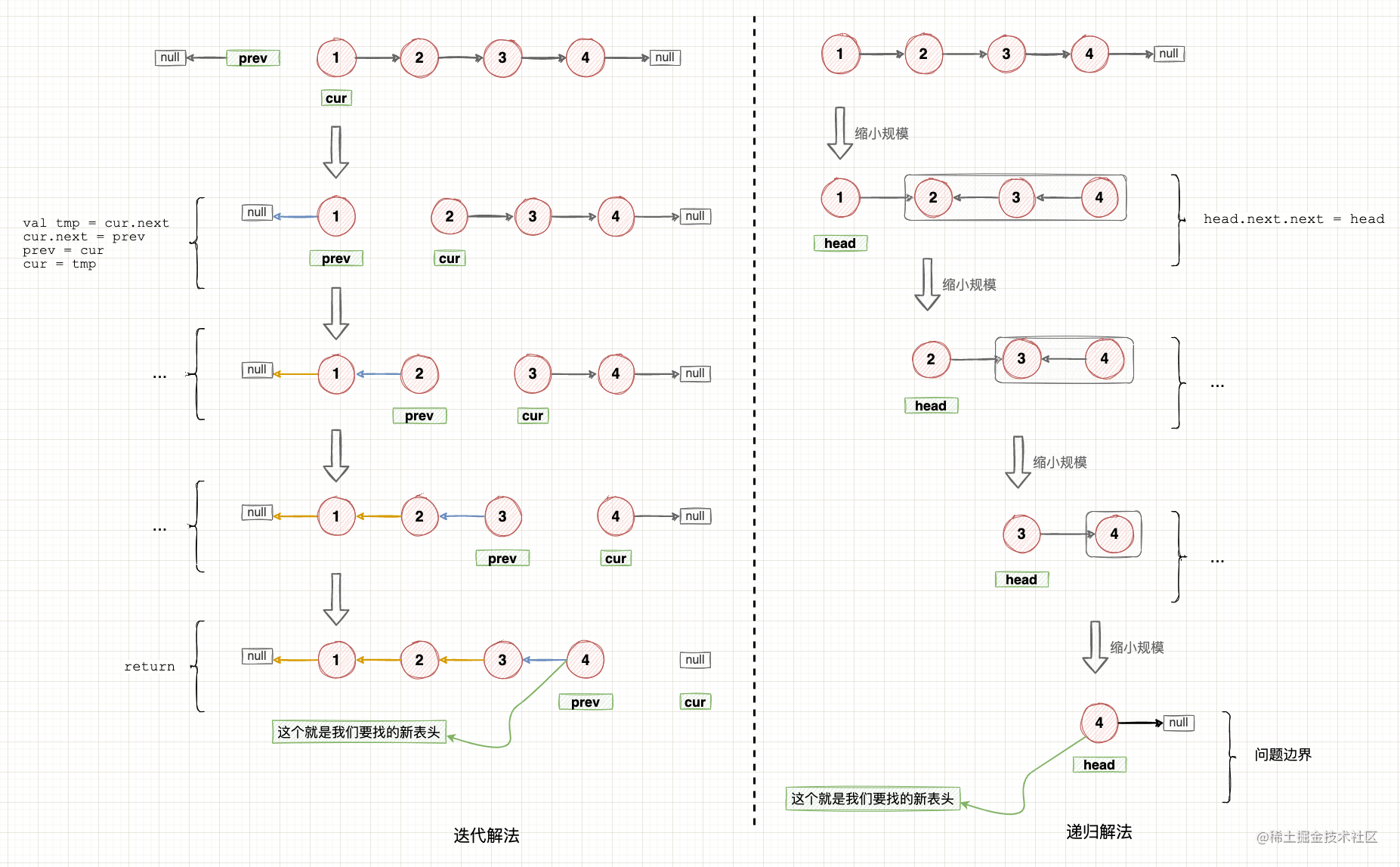迭代题解

class Solution {
fun reverseList(head: ListNode?): ListNode? {
var cur: ListNode? = head
var prev: ListNode? = null

while (null != cur) {
val tmp = cur.next
cur.next = prev
prev = cur
cur = tmp
}
return prev
}
}

• 时间复杂度：每个节点扫描一次，时间复杂度为 $O(n)$
• 空间复杂度：使用了常量级别变量，空间复杂度为 $O(1)$

递归题解

class Solution {
fun reverseList(head: ListNode?): ListNode? {
if(null == head || null == head.next){
}
}
}

• 时间复杂度：每个节点扫描一次，时间复杂度为 $O(n)$
• 空间复杂度：使用了函数调用栈，空间复杂度为 $O(n)$

# 5. 尾递归

尾递归

fun printList(itr : Iterator<*>){
if(!itr.hasNext()) {
return
}
println(itr.next())
// 尾递归
printList(itr)
}

迭代

fun printList(itr : Iterator<*>){
while(true) {
if(!itr.hasNext()) {
return
}
println(itr.next())
}
}

# 6. 总结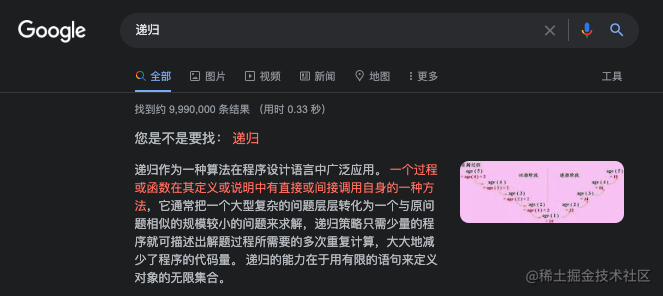### 参考资料Android